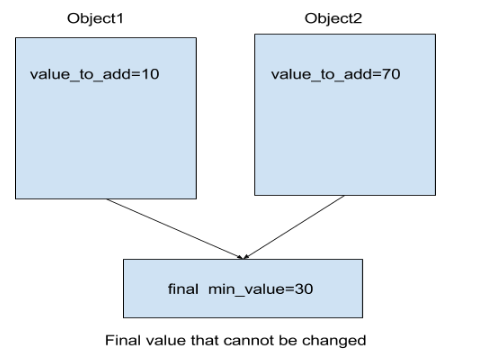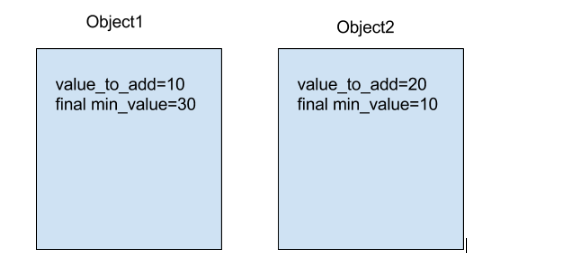# Java | static variables, instance variables, final and static

Author - Komal
19.01.2017
|
|

Explain with an example which variables should become instance variables and which others should become static. Also when to use final with static and final without static? (JAVA)

INSTANCE VARIABLES: Variables that belong to the object of the class and each object can have its own value for such a variable is known as instance variable (instance here means object).

Each object of that class has its own value for each instance variable
Instance variables takes memory in each object
Instance variables can only be used with the object of that class

For Example:

```Class Example{
int instance_variable;
}```

Calling:

```Example example_object=new Example();
example_object.instance_variable=10;```

STATIC VARIABLES: Static variables are the variables that belong to the class not the objects of that class.

There is only one value of that variable as there is a single copy of this variable.
Static variables are shared among all the objects.
It takes memory in class area.
There is no need to create the object of the class to use its static variable.

For Example:

```Class MyExample{
static int static_variable;
}```

Calling:

```MyExample.static_variable=10;
```

Which variables should become instance variables and which ones should become static
If we want to define a class member that can exist independently of any object of that class then static variable should be used. Instance variables should be used when the variable should be dependent on the object of that class.

The variables whose value will be different for each object should be defined as instance variables and the variables whose value will be same for each object should be defined as a static variable in order to save the memory.

For Example:

```Class Vehicle {
int vin; //instance variable since each car will have its own vin number
static int no_of_vehicles; //count of objects, independent of the object itself
Vehicle(int vin) {
this.vin = vin;
no_of_vehicle++;
}
public static getTotalVehicles() {
//static method for static variable
System.out.println(‘Total vehicles are’ + no_of_vehicle);
}
}
Class MainClass {
public static void main(String args[]) {
Vehicle object1 = new Vehicle(123456);
Vehicle object2 = new Vehicle(128756);
Vehicle.getTotalVehicles();
}
}```

Output:

Total vehicles are 2
When to use final with static and final without static

If there is a variable which can have different value for each object but once value is assigned it will not change then such a variable is a good fit for becoming final variable without static keyword. If there is a variable whose value will not change once assigned and values is also the same for every object, then it is a good fit for a final variable with static keyword.

For Example:

```Class FinalWithStatic {
final static int min_value = 30; //final with static
}
public int calculate() {
}
public static void main(String args[]) {
FinalWithStatic object1 = new FinalWithStatic(3);
FinalWithStatic object2 = new FinalWithStatic(70);
FinalWithStatic.min_value = 7; //ERROR final variable cannot be reinitialised
}

}``````Class FinalWithoutStatic {
final int min_value; //final but not static
this.min_value = min_value;
}
public int calculate() {